## 一种新型介电弹性体仿生可调焦透镜的变焦分析1)

*浙江大学航空航天学院工程力学系,杭州 310027

## THEORETICAL NONLINEAR ANALYSIS OF A BIOMIMETIC TUNABLE LENS DRIVEN BY DIELECTRIC ELASTOMER 1)

Shi Huiqi*, Wang Huiming,*,,2)

*Department of Engineering Mechanics,Zhejiang University,Hangzhou 310027, China

Key Laboratory of Soft Machines and Smart Devices of Zhejiang Province,Zhejiang University, Hangzhou 310027, China

 基金资助: 1) 国家自然科学基金资助项目.  11772296

Received: 2020-06-18   Accepted: 2020-09-7   Online: 2020-11-18Abstract

Dielectric elastomer (DE) is a class of electroactive polymer smart materials. Under the external electric field, it can produce various forms of responses. Comparing with the traditional lens with which the focus length is manipulated by the mechanical controls, the DE soft tunable lenses exhibit the distinct advantages in the tuning way of the focal length. The DE soft tunable lenses tune the focal length by mimicking the eyeball of human beings. The lens is composed of two circular DE films which are fixed on the rigid frame. The salty water is filled in the enclosed space and forms a convex lens. The top DE film is coated by the annular compliant electrodes. Under the voltage excitation, the upper film is deformed. Accordingly, the lower film is deformed due to the incompressibility of the salt water sealed in the enclosed space. Subsequently, the focal length of the tunable lens is changed. By employing the variational principle and the neo-Hookean model, we obtain the governing equations, boundary conditions and the continuity conditions of the biomimetic lens when driven by the dielectric elastomers. The nonlinear governing equations are solved by the shooting method and the continuity conditions at the interface are treated with in an effective way. The theoretical results agree well with the experimental data. The extensive parametric analysis is carried out based on the presented model. The numerical results show that the geometrical configuration, the initial focal length, the area of the coated annular compliant electrodes, the pre-stretch of the top DE film and the shear modulus of the bottom film have significant effect on the adjusting performance of the tunable lens. The presented theoretical model provides an effective tool in designing and optimizing the biomimetic adaptive focus lens.

Keywords： dielectric elastomers ; soft tunable lens ; focal length ; nonlinearity

Shi Huiqi, Wang Huiming. THEORETICAL NONLINEAR ANALYSIS OF A BIOMIMETIC TUNABLE LENS DRIVEN BY DIELECTRIC ELASTOMER 1). Chinese Journal of Theoretical and Applied Mechanics[J], 2020, 52(6): 1719-1729 DOI:10.6052/0459-1879-20-212

## 引言

### 图1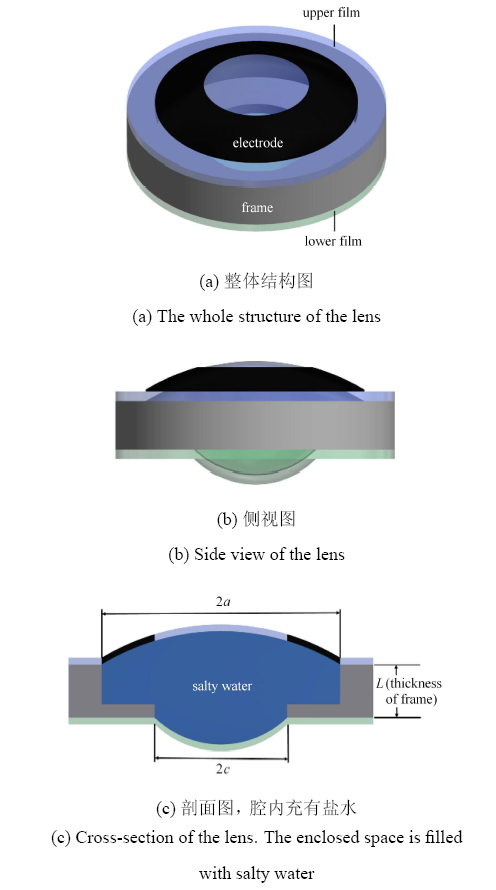Fig. 1   Schematics of the biomimetic dielectric elastomer adaptive focus lens (The upper and lower dielectric elastomer films are fixed on frames with radius $a$ and $c$, respectively. The upper film is coated with an annular electrode)

## 1 力学模型的建立

### 图2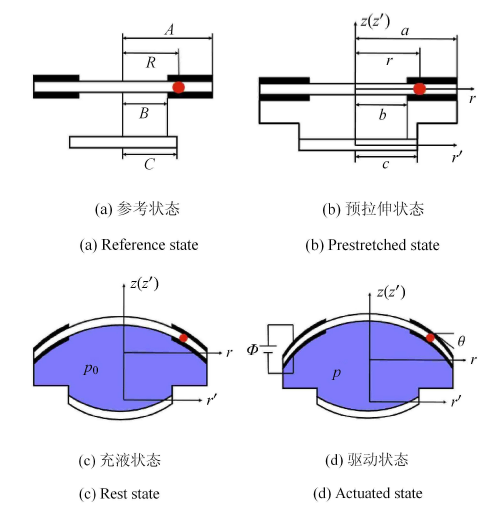Fig. 2   Mechanical model of biomimetic dielectric elastomer tunable lens (In each state, the position of a particular material particle is identified by a red dot. $r$-$z$ and $r'$-$z'$ are the coordinate systems of the upper film and the lower film in the current configuration, respectively)

$\dfrac{\text{d}r}{\text{d}R} = \lambda_1 \cos\theta$
$\dfrac{\text{d}z}{\text{d}R} = - \lambda_1 \sin\theta$

$V = \int_0^a 2\pi zr \text{d}r = \int_0^A 2\pi zr\dfrac{\text{d}r}{\text{d}R} \text{d}R$

$Q = 2\pi \int_B^A \tilde {D}R \text{d}R$

$W = W\left( {\lambda_1,\lambda_2,\tilde {D}} \right)$

$\delta W = s_1\delta \lambda_1 + s_2\delta \lambda_2 + \tilde {E}\delta \tilde {D}$

$s_1 = \dfrac{\partial W\left( {\lambda_1,\lambda_2,\tilde {D}} \right)}{\partial\lambda_1}$
$s_2 = \dfrac{\partial W\left( {\lambda_1,\lambda_2,\tilde {D}}\right)}{\partial \lambda_2}$
$\tilde {E} = \dfrac{\partial W\left( {\lambda_1,\lambda_2,\tilde {D}}\right)}{\partial \tilde {D}}$

$\dfrac{\text{d}}{\text{d}R}(HRs_1 \sin\theta ) - \lambda_1\lambda_2Rp \cos\theta = 0$
$\dfrac{\text{d}}{\text{d}R}(HRs_1 \cos\theta ) + \lambda_1\lambda_2Rp \sin\theta = s_2H$

$r^{(1)}(0) = 0 , \ \ \theta ^{(1)}(0) = 0$

$r^{(2)}(A) = a , \ \ z^{(2)}(A) = 0$

$\left.\begin{array}{l} r^{(1)}(B) = r^{(2)}(B) , \ \ z^{(1)}(B) = z^{(2)}(B) \\ \theta ^{(1)}(B) = \theta ^{(2)}(B) , \ \ s_1^{(1)} (B) = s_1^{(2)} (B) \end{array}\!\!\right\}$

$\left.\begin{array}{l} r '(0) = 0 , \ \ \theta '(0) = 0 \\ r '(C) = c , \ \ z '(C) = 0 \end{array}\!\!\right\}$

## 2 本构关系

$\tilde {E} = \dfrac{\tilde {D}}{\varepsilon }\lambda _1^{ - 2} \lambda _2^{ - 2}$

$W\left( {\lambda_1,\lambda_2,\tilde {D}} \right) = W_{stretch} \left( {\lambda_1,\lambda_2} \right) + \dfrac{\tilde {D}^2}{2\varepsilon }\lambda _1^{ - 2} \lambda _2^{ - 2}$

$W_{stretch} (\lambda_1,\lambda_2) = \dfrac{\mu }{2}(\lambda _1^2 + \lambda _2^2 + \lambda _1^{ - 2} \lambda _2^{ - 2} - 3)$

$s_1 = \mu \left( {\lambda _1 - \lambda _1^{ - 3} \lambda _2^{ - 2} } \right) -\varepsilon \tilde {E}^2\lambda _1 \lambda _2^2$
$s_2 = \mu \left( {\lambda _2 - \lambda _1^{ - 2} \lambda _2^{ - 3} } \right) - \varepsilon \tilde {E}^2\lambda _1^2 \lambda _2$

$\sigma _1 = \mu \left( {\lambda _1^2 - \lambda _1^{ - 2} \lambda _2^{ - 2} } \right) - \varepsilon E^2$
$\sigma _2 = \mu \left( {\lambda _2^2 - \lambda _1^{ - 2} \lambda _2^{ - 2} } \right) - \varepsilon E^2$

## 3 计算实现

$\dfrac{\text{d}\theta }{\text{d}R} = - \dfrac{s_2 }{s_1 }\dfrac{\sin\theta }{R} + \dfrac{\lambda _1 \lambda _2 }{s_1 }\dfrac{p}{H}$
$\dfrac{\text{d}\lambda _1 }{\text{d}R} = \dfrac{s_2 \cos\theta - s_1 + \dfrac{\text{d}s_1 }{\text{d}\lambda _2 }(\lambda _2 - \lambda _1 \cos\theta )}{R\dfrac{\text{d}s_1 }{\text{d}\lambda _1 }}$

$\dfrac{1}{f} = (n - 1)\left[ {\dfrac{1}{R_1 } - \dfrac{1}{R_2 } + \dfrac{(n - 1)d}{nR_1 R_2 }} \right]$

## 4 结果讨论

Table 1  Materials and parameters of the lens used in numerical simulation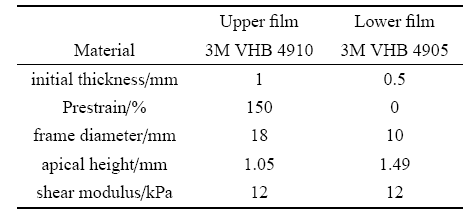### 图3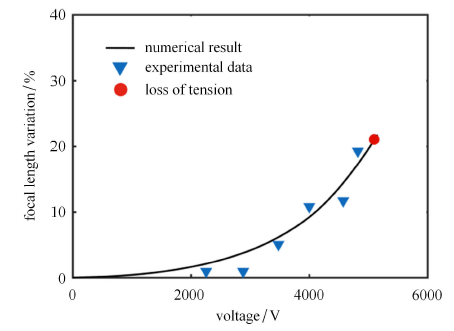Fig. 3   The comparison between the numerical results and the experimental data from Ref. (The filled triangles denote the experimental data. The solid circle corresponds to loss of tension)

### 图4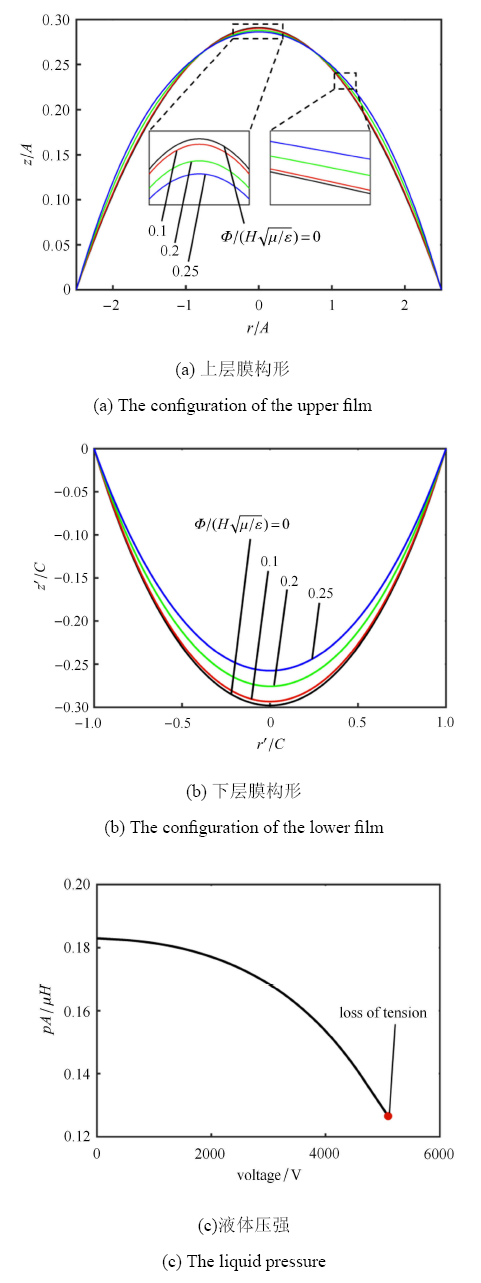Fig. 4   The configuration of the upper and lower films and the liquid pressure as a function of voltage

### 图5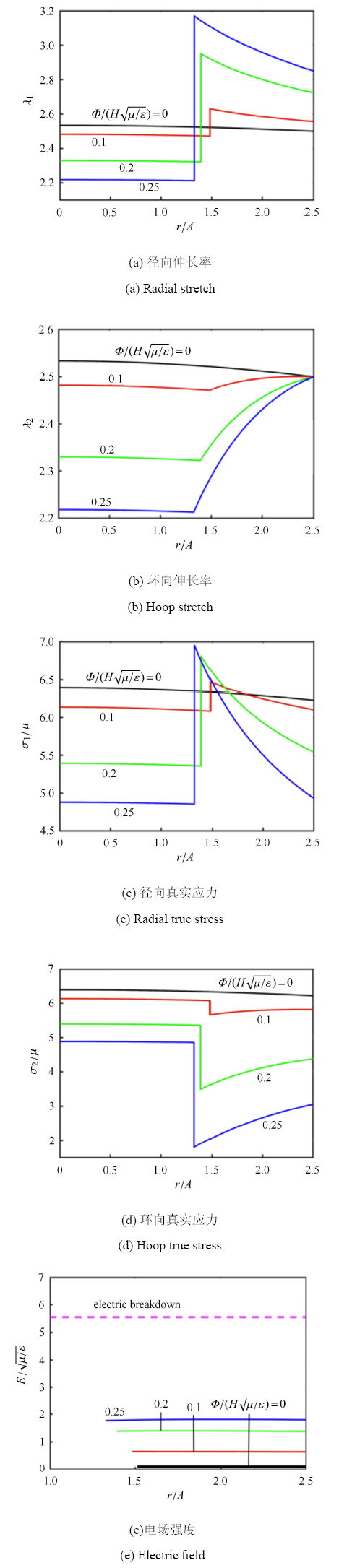Fig. 5   Distributions of various quantities in the upper film with inhomogeneous deformation

### 图6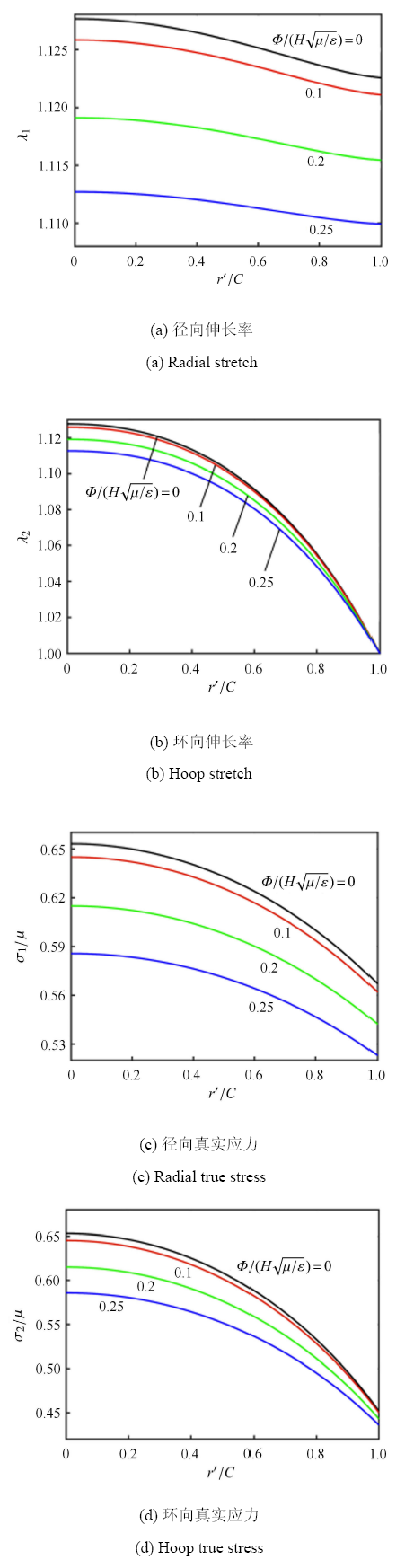Fig. 6   Distributions of various quantities in the lower film with inhomogeneous deformation

### 图7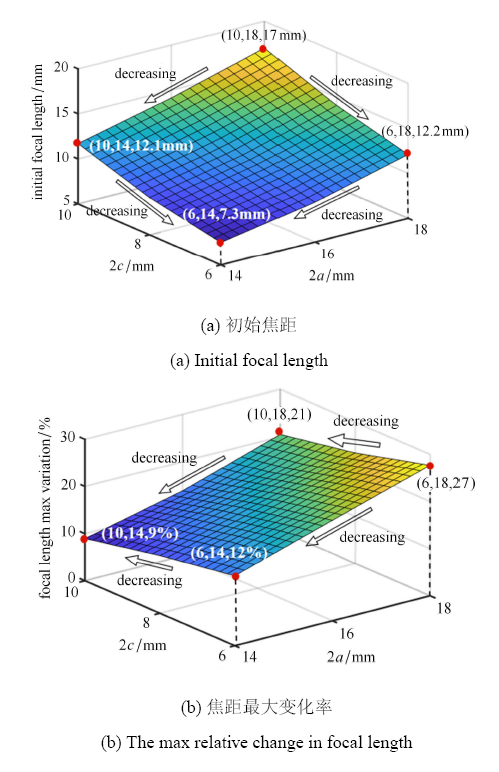Fig. 7   Changes in optical properties of the DE soft tunable lenses

### 图8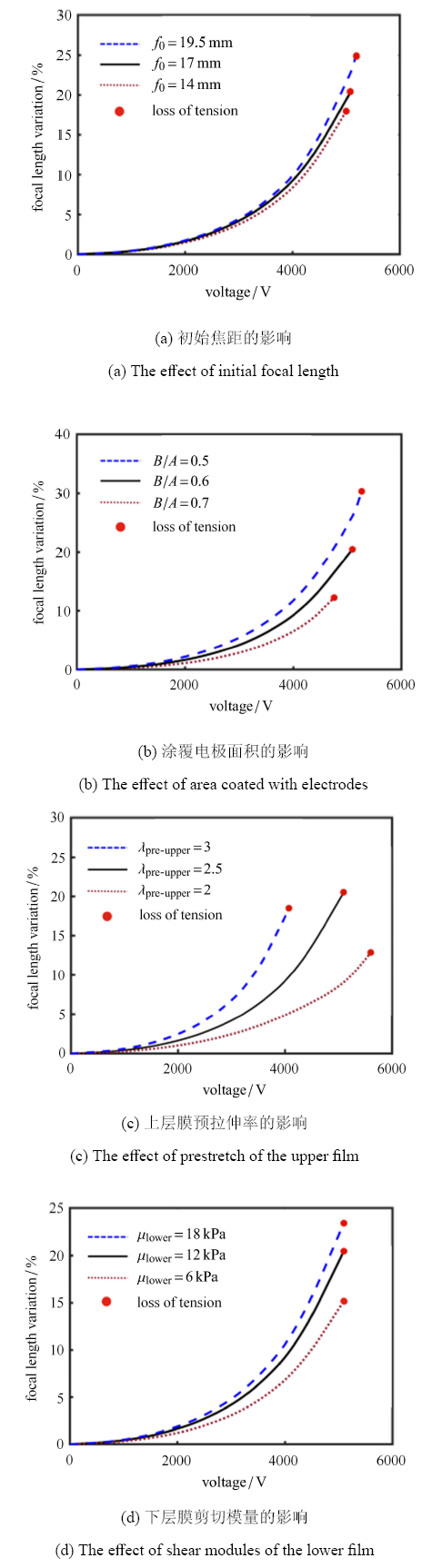Fig. 8   Parametric analysis of the DE soft tunable lenses

## 附录

$2\pi H\left[ {\int_0^B \delta W\left( {\lambda _1^{(1)} ,\lambda _2^{(1)} } \right)R \text{d}R + \int_B^A \delta W\left( {\lambda _1^{(1)} ,\lambda _2^{(1)} ,\tilde {D}} \right)R \text{d}R } \right] = p\delta V + \varPhi \delta Q$

$\delta \lambda _1^{(i)} = \dfrac{\text{d}\delta r^{(i)}}{\text{d}R}\cos \theta ^{(i)} - \dfrac{\text{d}\delta z^{(i)}}{\text{d}R}\sin \theta ^{(i)} \ \ \left( {i = 1,2} \right)$

$\delta \lambda _2^{(i)} = \dfrac{\delta r^{(i)}}{R}$

$\begin{array}{l} \int_0^B {\delta W\left( {\lambda _1^{(1)} ,\lambda _2^{(1)} } \right)} R \text{d}R =\\ s_1^{(1)} R\cos \theta ^{(1)}\delta r^{(1)}\vert _0^B - s_1^{(1)} R\sin \theta ^{(1)}\delta z^{(1)}\vert _0^B +\\ \int_0^B {\left( {s_2^{(1)} - \dfrac{\text{d}\left( {s_1^{(1)} R\cos \theta^{(1)}} \right)}{ \text{d}R }} \right)} \delta r^{(1)} \text{d}R +\\ \int_0^B {\dfrac{\text{d}\left( {s_1^{(1)} R\sin \theta ^{(1)}} \right)}{ \text{d}R }} \delta z^{(1)} \text{d}R \end{array}$
$\begin{array}{l}\int_B^A {\delta W\left( {\lambda _1^{(1)} ,\lambda _2^{(1)} ,\tilde {D}} \right)} R \text{d}R =\\ s_1^{(2)} R\cos \theta ^{(2)}\delta r^{(2)}\vert _B^A - s_1^{(2)} R\sin\theta ^{(2)}\delta z^{(2)}\vert _B^A +\\ \int_B^A {\left( {s_2^{(2)} - \dfrac{\text{d}\left( {s_1^{(2)} R\cos \theta^{(2)}} \right)}{ \text{d}R }} \right)} \delta r^{(2)} \text{d}R +\\ \int_B^A {\dfrac{d\left( {s_1^{(2)} R\sin \theta ^{(2)}} \right)}{ \text{d}R }} \delta z^{(2)} \text{d}R + \int_B^A {\tilde {E}\delta \tilde {D}} R \text{d}R \end{array}$

$\begin{array}{l} p\delta V = 2\pi pr^{(1)}z^{(1)}\delta r^{(1)}\vert _0^B - 2\pi pr^{(2)}z^{(2)}\delta r^{(2)}\vert _B^A+\\ 2\pi p\int_0^B {\left( {r^{(1)}\dfrac{\text{d}r^{(1)}}{\text{d}R }\delta z^{(1)} - r^{(1)}\dfrac{\text{d}z^{(1)}}{ \text{d}R }\delta r^{(1)}} \right)} \text{d}R +\\ 2\pi p\int_B^A {\left( {r^{(2)}\dfrac{\text{d}r^{(2)}}{ \text{d}R }\delta z^{(2)} - r^{(2)}\dfrac{\text{d}z^{(2)}}{ \text{d}R }\delta r^{(2)}} \right)} \text{d}R \end{array}$

$\varPhi \delta Q = 2\pi \int_B^A {\varPhi \delta \tilde {D}R \text{d}R}$

$\dfrac{\text{d}}{\text{d}R}(HRs_{1}^{(i)} \sin\theta ^{(i)}) - \lambda _{1}^{(i)} \lambda_{2}^{(i)} Rp\cos\theta ^{(i)} = 0$
$\dfrac{\text{d}}{\text{d}R}(HRs_{1}^{(i)} \cos\theta ^{(i)}) + \lambda _{1}^{(i)} \lambda_{2}^{(i)} Rp\sin\theta ^{(i)} = s_{2}^{(i)} H$
$\tilde {E} = \varPhi / H$

$s_1^{(1)} \cos \theta ^{(1)} = s_1^{(2)} \cos \theta ^{(2)}$
$s_1^{(1)} \sin \theta ^{(1)} = s_1^{(2)} \sin \theta ^{(2)}$

$\theta ^{(1)}(B) = \theta ^{(2)}(B) ,\quad s_1^{(1)} (B) = s_1^{(2)} (B)$

## 参考文献 原文顺序 文献年度倒序 文中引用次数倒序 被引期刊影响因子

Friese C, Werber A, Krogmann A, et al.

Materials, effects and components for tunable micro-optics

IEEJ Transactions on Electrical and Electronic Engineering, 2007,2(3):232-248

Dong L, Agarwal AK, Beebe DJ, et al.

Adaptive liquid microlenses activated by stimuli-responsive hydrogels

Nature, 2006,442(7102):551-554

URL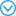Despite its compactness, the human eye can easily focus on different distances by adjusting the shape of its lens with the help of ciliary muscles. In contrast, traditional man-made optical systems achieve focusing by physical displacement of the lenses used. But in recent years, advances in miniaturization technology have led to optical systems that no longer require complicated mechanical systems to tune and adjust optical performance. These systems have found wide use in photonics, displays and biomedical systems. They are either based on arrays of microlenses with fixed focal lengths, or use external control to adjust the microlens focal length. An intriguing example is the tunable liquid lens, where electrowetting or external pressure manipulates the shape of a liquid droplet and thereby adjusts its optical properties. Here we demonstrate a liquid lens system that allows for autonomous focusing. The central component is a stimuli-responsive hydrogel integrated into a microfluidic system and serving as the container for a liquid droplet, with the hydrogel simultaneously sensing the presence of stimuli and actuating adjustments to the shape--and hence focal length--of the droplet. By working at the micrometre scale where ionic diffusion and surface tension scale favourably, we can use pinned liquid-liquid interfaces to obtain stable devices and realize response times of ten to a few tens of seconds. The microlenses, which can have a focal length ranging from -infinity to +infinity (divergent and convergent), are also readily integrated into arrays that may find use in applications such as sensing, medical diagnostics and lab-on-a-chip technologies.

Li GQ, Mathine DL, Valley P, et al.

Switchable electro-optic diffractive lens with high efficiency for ophthalmic applications

Proceedings of the National Academy of Sciences of the United States of America, 2006,103(16):6100-6104

Wang WS, Fang J.

Design, fabrication and testing of a micromachined integrated tunable microlens

Journal of Micromechanics and Microengineering, 2006,16(7):1221-1226

Choi D, Jeong J, Shin E, et al.

Focus-tunable double convex lens based on non-Ionic electroactive gel

Optics Express, 2017,25(17):20133-20141

URLWe propose a focus-tunable double-convex (DCX) lens based on a non-ionic PVC (nPVC) gel to be used at close conjugates. The proposed lens is composed of an nPVC gel and two plates with electrodes. Each plate has a hole whose boundary and inner part are pasted with an electrode (anode) and has another ring shaped electrode (cathode) whose center point is the same as the hole's center. The gel is sandwiched between an upper plate and a lower plate, and it is bulged inward between the holes of two plates by applied pressure from the plates (double-convex lens shape). The lens's focal length changed from 3 mm to 24.5 mm with applied voltages from 0 V to 400 V. We also observed that the proposed lens's field-of-view decreased from 121.9 degrees to 41.9 degrees according to the applied voltages. The proposed lens brings additional benefit for users with higher transmittance (over 94%).

( Jia Shuhai, Tang Zhenhua, Dong Jun, et al.

Recent advances in flexible variable-focus lens

Chinese Optics and Applied Optics Abstracts, 2015,8(4):535-547 (in Chinese))

( Zou Yi, Li Hua, Cao Yang, et al.

Variable-focus liquid lens using frequency control

Journal of Suzhou University of Science and Technology (Natural Science Edition), 2017,34(3):45-49 (in Chinese))

Gowda HGB, Wallrabe U.

Simulation of an adaptive fluid-membrane piezoelectric lens

Micromachines, 2019,10(12):797

Huang X, Jin H, Lin SY, et al.

Optics Letters, 2020,45(2):331-334

Song XM, Zhang HX, Li DY, et al.

Liquid lens with large focal length tunability fabricated in a polyvinyl chloride/dibutyl phthalate gel tube

Langmuir, 2020,36(6):1430-1436

URLUsually, an adaptive liquid lens only has a positive focal length, which severely limits its application in imaging and other fields. Therefore, a liquid lens consisting of polyvinyl chloride/dibutyl phthalate (PVC/DBP) gel, glycerol solution, and a glass substrate is proposed to extend the dynamic focal length range. A spherical tube is formed by the PVC/DBP gel under the effect of hydrostatic and surface tensions, which is used to restrict the glycerol solution. The PVC/DBP gel does not deform under the effect of an electric field, so the tangent line at the three-phase junction changes with the change of contact angle, which leads to an enlargement of the dynamic focal length range. At different voltage values, the proposed lens can be configured to work in three different schemes, namely, converging light, nondeflecting light, and diverging light. Here, the proposed lens has high imaging quality; the resolution is better than 114 lp/mm. A lens with a reconfigurable focal length holds great promise in diverse applications such as fluorescence detection, beam shaping, and adaptive optics.

Ghilardi M, Boys H, Torok P, et al.

Smart lenses with electrically tuneable astigmatism

Scientific Reports, 2019,9(1):16127

URLThe holy grail of reconfigurable optics for microscopy, machine vision and other imaging technologies is a compact, in-line, low cost, refractive device that could dynamically tune optical aberrations within a range of about 2-5 wavelengths. This paper presents the first electrically reconfigurable, fully elastomeric, tuneable optical lenses with motor-less electrical controllability of astigmatism in the visible range. By applying different voltage combinations to thin dielectric elastomer actuator segments surrounding a soft silicone lens, we show that the latter can be electrically deformed either radially or along selectable directions, so as to tune defocus or astigmatism, up to about 3 wavelengths. By mounting the new lenses on a commercial camera, we demonstrate their functionality, showing how electrically reconfiguring their shape can be used to dynamically control directional blurring while taking images of different targets, so as to emphasize directional features having orthogonal spatial orientations. Results suggest that the possibility of electrically controlling aberrations inherent to these smart lenses holds promise to develop highly versatile new components for adaptive optics.

Huang HY, Zhao Y.

Optofluidic lenses for 2D and 3D imaging

Journal of Micromechanics and Microengineering, 2019,29(7):073001

Mockensturm EM, Goulbourne N.

Dynamic response of dielectric elastomers

International Journal of Non-Linear Mechanics, 2006,41(3):388-395

Plante JS, Dubowsky S.

Large-scale failure modes of dielectric elastomer actuators

International Journal of Solids and Structures, 2006,43(25):7727-7751

Patrick L, Gabor K, Silvain M.

Characterization of dielectric elastomer actuators based on a visco-hyperelastic film model

Smart Materials and Structures, 2007,16(2):477-486

Koh KH, Sreekumar M, Ponnambalam SG.

Hybrid electrostatic and elastomer adhesion mechanism for wall climbing robot

Mechatronics, 2016,35:122-135

Shian S, Bertoldi K, Clarke DR.

Dielectric elastomer based "grippers" for soft robotics

Gupta U, Qin L, Wang YZ, et al.

Soft robots based on dielectric elastomer actuators: A review

Smart Materials and Structures, 2019,28(10):103002

Bortot E, Springhetti R, de Botton G, et al.

Optimization of load-driven soft dielectric elastomer generators

Procedia IUTAM, 2015,12:42-51

McKay TG, Rosset S, Anderson IA, et al.

Dielectric elastomer generators that stack up

Smart Materials and Structures, 2015,24(1):015014

Carpi F, Frediani G, Turco S, et al.

Bioinspired tunable lens with muscle-like electroactive elastomers

URLOptical lenses with tunable focus are needed in several fields of application, such as consumer electronics, medical diagnostics and optical communications. To address this need, lenses made of smart materials able to respond to mechanical, magnetic, optical, thermal, chemical, electrical or electrochemical stimuli are intensively studied. Here, we report on an electrically tunable lens made of dielectric elastomers, an emerging class of "artificial muscle" materials for actuation. The optical device is inspired by the architecture of the crystalline lens and ciliary muscle of the human eye. It consists of a fluid-filled elastomeric lens integrated with an annular elastomeric actuator working as an artificial muscle. Upon electrical activation, the artificial muscle deforms the lens, so that a relative variation of focal length comparable to that of the human lens is demonstrated. The device combined optical performance with compact size, low weight, fast and silent operation, shock tolerance, no overheating, low power consumption, and possibility of implementation with inexpensive off-the-shelf elastomers. Results show that combing bioinspired design with the unique properties of dielectric elastomers as artificial muscle transducers has the potential to open new perspectives on tunable optics.

Zhang H, Dai M, Zhang ZS.

The analysis of transparent dielectric elastomer actuators for lens

Optik, 2019,178:841-845

She A, Zhang SY, Shian S, et al.

Adaptive metalenses with simultaneous electrical control of focal length, astigmatism, shift

Suo ZG.

Theory of dielectric elastomers

Acta Mechanica Solida Sinica, 2010,23(6):549-578

URLAbstractIn response to a stimulus, a soft material deforms, and the deformation provides a function. We call such a material a soft active material (SAM). This review focuses on one class of soft active materials: dielectric elastomers. When a membrane of a dielectric elastomer is subject to a voltage through its thickness, the membrane reduces thickness and expands area, possibly straining over 100%. The dielectric elastomers are being developed as transducers for broad applications, including soft robots, adaptive optics, Braille displays, and electric generators. This paper reviews the theory of dielectric elastomers, developed within continuum mechanics and thermodynamics, and motivated by molecular pictures and empirical observations. The theory couples large deformation and electric potential, and describes nonlinear and nonequilibrium behavior, such as electromechanical instability and viscoelasticity. The theory enables the finite element method to simulate transducers of realistic configurations, predicts the efficiency of electromechanical energy conversion, and suggests alternative routes to achieve giant voltage-induced deformation. It is hoped that the theory will aid in the creation of materials and devices.]]>

( Liu Yanju, Liu Liwu, Sun Shouhua, et al.

Stability analysis of dielectric elastomer film actuator

Science in China (Series E), 2009,39:1564-1573 (in Chinese))

Lu TQ, Ma C, Wang TJ.

Mechanics of dielectric elastomer structures: A review

Extreme Mechanics Letters, 2020,38:100752

( Wei Zhigang, Chen Haibo.

A new elastic model for rubber-like materials

Chinese Journal of Theoretical and Applied Mechanics, 2019,51(2):473-483 (in Chinese))

Li JR, Wang Y, Liu LW, et al.

A biomimetic soft lens controlled by electrooculographic signal

Pelrine RE, Kornbluh RD, Pei QB, et al.

High-speed electrically actuated elastomers with strain greater than 100%

Science, 2000,287(5454):836-839

URLElectrical actuators were made from films of dielectric elastomers (such as silicones) coated on both sides with compliant electrode material. When voltage was applied, the resulting electrostatic forces compressed the film in thickness and expanded it in area, producing strains up to 30 to 40%. It is now shown that prestraining the film further improves the performance of these devices. Actuated strains up to 117% were demonstrated with silicone elastomers, and up to 215% with acrylic elastomers using biaxially and uniaxially prestrained films. The strain, pressure, and response time of silicone exceeded those of natural muscle; specific energy densities greatly exceeded those of other field-actuated materials. Because the actuation mechanism is faster than in other high-strain electroactive polymers, this technology may be suitable for diverse applications.

Lu TQ, Cai SQ, Wang HM, et al.

Computational model of deformable lenses actuated by dielectric elastomers

Journal of Applied Physics, 2013,114(10):104104

Wang HM, Cai SQ, Carpi F, et al.

Computational model of hydrostatically coupled dielectric elastomer actuators

Journal of Applied Mechanics, 2012,79(3):031008

Li JR, Lv XF, Liu LW, et al.

Computational model and design of the soft tunable lens actuated by dielectric elastomer

Journal of Applied Mechanics, 2020,87(7):071005

( Yang Jianpeng, Wang Huiming.

Chemomechanical analysis of a functionally graded spherical hydrogel

Chinese Journal of Theoretical and Applied Mechanics, 2019,51(4):1054-1063 (in Chinese))

( Zheng Baojing, Liang Yu, Gao Xiaowei, et al.

Analysis for dynamic response of functionally graded materials using pod based reduced order model

Chinese Journal of Theoretical and Applied Mechanics, 2018,50(4):787-797 (in Chinese))

Zhao XH, Hong W, Suo ZG.

Electromechanical hysteresis and coexistent states in dielectric elastomers

Physical Review B, 2007,76(13):134113

Shian S, Diebold RM, Clarke DR.

Tunable lenses using transparent dielectric elastomer actuators

Optics Express, 2013,21(7):8669-8676

URLFocus tunable, adaptive lenses provide several advantages over traditional lens assemblies in terms of compactness, cost, efficiency, and flexibility. To further improve the simplicity and compact nature of adaptive lenses, we present an elastomer-liquid lens system which makes use of an inline, transparent electroactive polymer actuator. The lens requires only a minimal number of components: a frame, a passive membrane, a dielectric elastomer actuator membrane, and a clear liquid. The focal length variation was recorded to be greater than 100% with this system, responding in less than one second. Through the analysis of membrane deformation within geometrical constraints, it is shown that by selecting appropriate lens dimensions, even larger focusing dynamic ranges can be achieved.

Li TF, Keplinger C, Baumgartner R, et al.

Giant voltage-induced deformation in dielectric elastomers near the verge of snap-through instability

Journal of the Mechanics and Physics of Solids, 2013,61(2):611-628

/

 〈〉